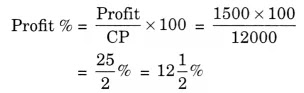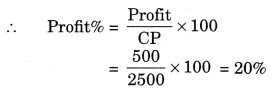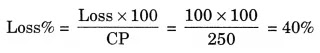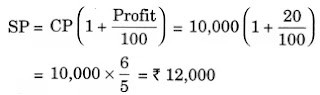NCERT Solutions for Class 7 Maths Chapter 8 Comparing Quantities Ex 8.3

# NCERT Solutions for Class 7 Maths Chapter 8 Comparing Quantities Ex 8.3

## NCERT Solutions for Class 7 Maths Chapter 8 Comparing Quantities Ex 8.3

NCERT Solutions for Class 7 Maths Chapter 8 Comparing Quantities Ex 8.3 are the part of NCERT Solutions for Class 7 Maths. Here you can find the NCERT Solutions for Class 7 Maths Chapter 8 Comparing Quantities Ex 8.3.

### Ex 8.3 Class 7 Maths Question 1.

Tell what is the profit or loss in the following transactions. Also find profit per cent or loss per cent in each case.
(a) Gardening shears bought for ₹ 250 and sold for ₹ 325.
(b) A refrigerator bought for ₹ 12,000 and sold at ₹ 13,500.
(c) A cupboard bought for ₹ 2,500 and sold at ₹ 3,000.
(d) A skirt bought for ₹ 250 and sold at ₹ 150.

Solution:
(a) Here, CP = ₹ 250 and SP = ₹ 325
Since, SP > CP, there is a profit.
Profit = SP – CP
= ₹ 325 – ₹ 250 = ₹ 75Hence, the required profit is ₹ 75 and profit per cent is 30%.

(b) Here, CP = ₹ 12,000 and SP = ₹ 13,500
Since, SP > CP, there is a profit.
Profit = SP – CP
= ₹ 13,500 – ₹ 12,000 = ₹ 1,500Hence, the required profit is ₹ 1500 and profit per cent is 121/2%.

(c) Here, CP = ₹ 2500 and SP = ₹ 3000
Since, SP > CP, there is a profit.
Profit = SP – CP
= ₹ 3000 – ₹ 2500 = ₹ 500Hence, the required profit is ₹ 500 and profit per cent is 20%.

(d) Here, CP = ₹ 250 and SP = ₹ 150
Since, SP < CP, there is a loss.
Loss = CP – SP
= ₹ 250 – ₹ 150 = ₹ 100Hence, the required loss is ₹ 100 and loss per cent is 40%.

### Ex 8.3 Class 7 Maths Question 2.

Convert each part of the ratio to percentage:
(a) 3 : 1
(b) 2 : 3 : 5
(c) 1 : 4
(d) 1 : 2 : 5

Solution:
(a) 3 : 1
Sum of the ratio parts = 3 + 1 = 4

(b) 2 : 3 : 5
Sum of the ratio parts = 2 + 3 + 5 = 10

(c) 1 : 4
Sum of the ratio parts = 1 + 4 = 5

(d) 1 : 2 : 5
Sum of the ratio parts = 1 + 2 + 5 = 8

### Ex 8.3 Class 7 Maths Question 3.

The population of a city decreased from 25,000 to 24,500. Find the percentage decrease.

Solution:
Initial population of the city = 25,000
Decreased population of city = 24,500
Decrease in population = 25,000 – 24,500 = 500
Percentage decrease = (500 × 100)/25000 = 2%
Hence, the percentage decrease in population of the city is 2%.

### Ex 8.3 Class 7 Maths Question 4.

Arun bought a car for ₹ 3,50,000. The next year, the price went up to ₹ 3,70,000. What was the percentage of price increase?

Solution:
Original price of the car = ₹ 3,50,000
Increased price of the car = ₹ 3,70,000
Increase in price = ₹ 3,70,000 – ₹ 3,50,000
= ₹ 20,000
Percentage increase

Hence, the percentage increase in the price of the car is 55/7 %.

### Ex 8.3 Class 7 Maths Question 5.

I buy a TV for ₹ 10,000 and sell it at a profit of 20%. How much money do I get for it?

Solution:
Here, CP = ₹ 10,000 and Profit = 20%
SP = ?Hence, the money got by me for the TV is ₹ 12,000.

### Ex 8.3 Class 7 Maths Question 6.

Juhi sells a washing machine for ₹ 13,500. She loses 20% in the bargain. What was the price at which she bought it?

Solution:
Here, Selling price of the washing machine = ₹ 13,500
Loss = 20%
CP = ?Hence, the cost price of the washing machine is ₹ 16,875.

### Ex 8.3 Class 7 Maths Question 7.

(i) Chalk contains calcium, carbon and oxygen in the ratio 10 : 3 : 12. Find the percentage of carbon in chalk.
(ii) If in a stick of chalk, carbon is 3 g, what is the weight of the chalk stick?

Solution:
(i) Sum of the ratio parts = 10 + 3 + 12 = 25
Percentage of carbon in chalk
= (3/25) × 100% = 12%
Hence, the percentage of carbon in chalk is 12%.

(ii) Weight of carbon = 3 g

If carbon part in chalk stick is 3, the total part is 25.

If carbon weight is 3 g, the total weight of the chalk stick = (3 × 25)/3 g = 25 g
Hence, the weight of chalk stick is 25 g.

### Ex 8.3 Class 7 Maths Question 8.

Amina buys a book for ₹ 275 and sells it at a loss of 15%. How much does she sell it for?

Solution:
Here, CP of the book = ₹ 275 and Loss = 15%

Hence, the selling price of the book is ₹ 233.75.

### Ex 8.3 Class 7 Maths Question 9.

Find the amount to be paid at the end of 3 years in each case.
(a) Principal = ₹ 1200 at 12% p.a.
(b) Principal = ₹ 7500 at 5% p.a.

Solution:
(a) Given: Principal (P) = ₹ 1200
Rate of interest (R) = 12% p.a., T = 3 years
Simple Interest = (P × R × T)/100 = (1200 × 12 × 3)/100 = ₹432
Amount = Principal + Interest
= ₹ 1200 + ₹ 432 = ₹ 1632
Hence, the required amount is ₹ 1632.

(b) Given: Principal (P) = ₹ 7500
Rate of interest (R) = 5% p.a., T = 3 years
Simple Interest = (P × R × T)/100 = (7500 × 5 × 3)/100 = ₹1125
Amount = Principal + Interest
= ₹ 7500 + 1125 = ₹ 8625
Hence, the required amount is ₹ 8625.

### Ex 8.3 Class 7 Maths Question 10.

What rate gives ₹ 280 as interest on a sum of ₹ 56,000 in 2 years?

Solution:
Given: Principal (P) = ₹ 56,000
Interest (I) = ₹280 and Time = 2 years
Rate of interest (R) = ?

Hence, the required rate of interest is 0.25%.

### Ex 8.3 Class 7 Maths Question 11.

If Meena gives an interest of ₹ 45 for one year at 9% rate p.a. What is the sum she has borrowed?

Solution:
Given: Interest (I) = ₹45
Time (T) = 1 year
Rate of interest (R) = 9% p.a.

Hence, the required sum she has borrowed is ₹ 500.

You can also like these:

NCERT Solutions for Maths Class 8

NCERT Solutions for Maths Class 9

NCERT Solutions for Maths Class 10

NCERT Solutions for Maths Class 11

NCERT Solutions for Maths Class 12